Next: Density functional theory Up: Quantum Mechanics Previous: Problems

### Wave packets and wave packet dynamics

wpdynamics -->

Wave packet dynamics

What is a wave packet?

In quantum mechanics the motion of a particle is represented by that of a wave packet. The wave packet is a wavefunction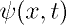obtained by superposing many harmonic (sine, cosine) or equivalently exp(ikx) wavefunctions  corresponding to different linear momenta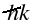. The larger the number of wave functions in the superposition, the more localized the wave packet is in space and the corresponding particle can be measured more precisely. Simultaneously the measurement of the momentum of the particle becomes more imprecise. This is in accordance with Heisenberg's uncertainty principle.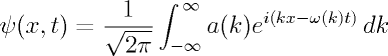The coefficients (a(k)) are obtained by taking the inverse Fourier transform of the superposed wave function at t=0 as follows:The wave functionis the most general solution of the time dependent Schrodinger equation for a free particle. The w-k relationship for such a particle obtained from the the deBroglie relations is found to be non-linear.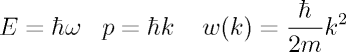Hence the wave packet for the free particle moving in such a dispersive medium would get temporally dispersed or distorted while evolving with time with a group velocity. k is centred about k_{0} and is assumed to vary little. It bears mention that an electromagnetic wave travelling in free space corresponds to a non-dispersive medium as the w-k relationship is linear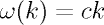c being the speed of light which is constant.  The wave packet then travels undistorted with constant speed c.

Simulation of a wave packet striking a potential barrier

Now we consider what happens when a particle encounters a rectangular potential barrier of infinite height and finite width. If the particle behaves classically, there is no way it can go over to the other side of the barrier. However, from a quantum mechanical perspective, the particle can be represented as a wave packet and the wave packet can be shown to penetrate or tunnel through the barrier. Below is presented a simulation  which makes it more understandable.

To obtain the simulation, one needs to solve Schrodinger's time dependent equation for the rectangular barrier potential. The solution may be launched with a Gaussian wave packet and the modulus of the wave function representing the wave packet is to be plotted at fixed intervals of time. Finally the plots are to be animated with the help of an image manipulation program like photoshop or gimp.

Hey, do you notice that along with the transmitted wave there are also a few reflected components?

Do you want to create such a simulation yourself? Refer to Goldberg et al.'s paper (Am. J. Phys., 35, 177 (1967)).

Abhijit Poddar
2007-09-27